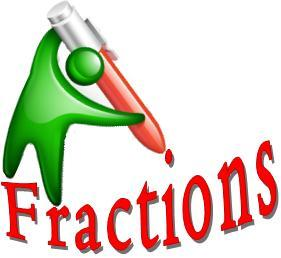# Do Year 5 Know Fractions?

20 Questions | Total Attempts: 302SettingsLet's test our knowledge! Are you ready for our Fraction Quiz? Can you identify which fraction is bigger? Which is improper? What about the equivalent of a fraction? This quiz will test your current knowledge of fractions. Let's see who our class geniuses are! Fractions aren't that hard so let's see how you do!

• 1.
What fraction of this figure is shaded?
• 2.
What fraction of this figure is NOT shaded?
• A.

5/8

• B.

3/4

• C.

3/8

• D.

1/2

• 3.
Fill in the blank:
• 4.
What Number in a fraction is the denominator?
• A.

The top number

• B.

The bottom number

• 5.
Fill in the blank:
• 6.
Describe this fraction as either proper, improper or mixed:
• 7.
What is 2/4 equal to?
• A.

2/2

• B.

1/2

• C.

1/4

• D.

4/2

• 8.
What is 2/6 equal to?
• A.

1/2

• B.

3/4

• C.

1/3

• D.

2/5

• 9.
What number is the numerator?
• A.

The top number

• B.

The bottom number

• 10.
If there are 4 pieces of cake and there used to be 6 what is the fraction represents the amount of cake left?
• A.

1/2

• B.

5/6

• C.

4/3

• D.

2/3

• 11.
Describe this fraction as either proper, improper or mixed:
• 12.
Describe this fraction as either proper, improper or mixed:
• 13.
​True or False:
• A.

True

• B.

False

• 14.
True or False:
• A.

False

• B.

True

• 15.
​How many 1/4's are in 1 3/4?
• 16.
Convert to an improper fraction:
• 17.
Convert to an improper fraction:
• 18.
Convert to a Mixed Number:
• 19.
Simplify this fraction:
• 20.
Simplify this fraction:
Related TopicsBack to top